# A canal runs through it

Colin Beveridge barges in and admires some curious railway bridges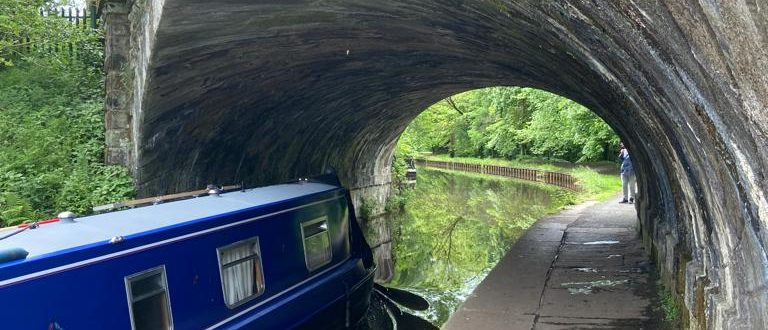For those of you who haven’t already switched, this article will mark a dividing point between two phases of your life: the before, when you would be able to simply walk under a railway bridge; and the after when you will feel compelled to stop and check.

Find your nearest arched railway bridge built out of bricks, the traditional type that looks like cuboids. Go ahead, I’ll be here when you get back. Found one? Great. The chances are, the bricks were in one of two configurations. Either they were laid in rows with the longest brick edges horizontal, as shown in the first photo below, or the rows were all slanted—set at a nonzero angle to the horizontal, as in the second photo.

Why would you ever build a bridge with bricks that aren’t horizontal? That’s an excellent question. Someone should write a Chalkdust article about that. While they’re doing it, they should also explain why these diagonal bricks have a suboptimal configuration, and how to do it properly.

Now, walls—walls are simple. Walls are practically always built with bricks in rows parallel to the ground because bricks are very strong in compression—they tend to hold up well if you squish them. By contrast, if you push two bricks past each other—ie if they undergo a shearing force—they are more likely to slip past each other in which case, the wall fails. Mortar can help to some extent, but it’s still significantly better to build walls so that the forces involved act perpendicular to the joins between the rows of bricks. If you build a wall with horizontal bricks, like this below,the forces on each brick all act vertically—neither the brick’s weight nor the contact forces with its neighbours in the rows above and below have any horizontal component. You could remove the mortar and the wall would be perfectly fine (unless something bumped into it). If, however, you build the wall with the long brick edges at an acute angle to the ground, like this,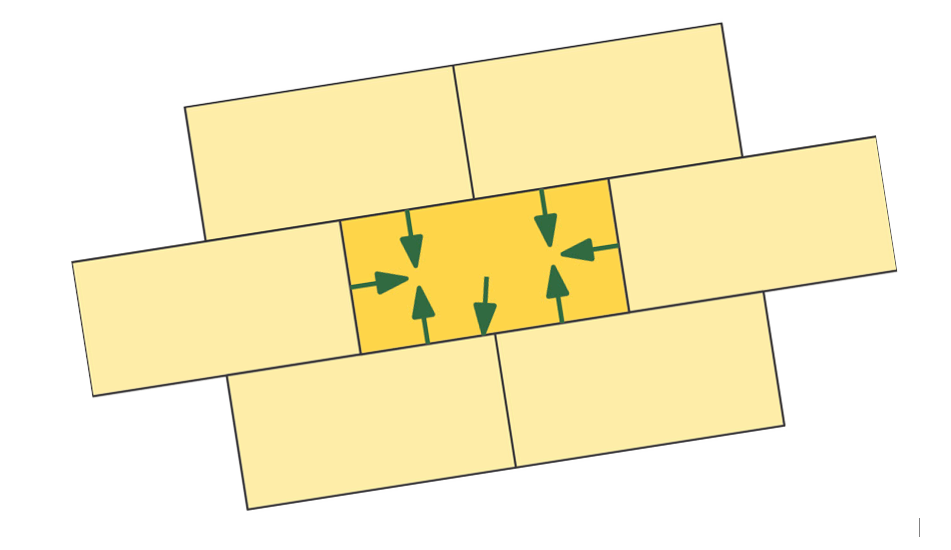suddenly the mortar becomes important because the rows of bricks have a tendency to slide past each other. Think about the brick at the lower end of the top row: without mortar, there’s nothing to stop it just sliding off, with the rest of the row following. Even with mortar, the extra shear forces make it more likely to fail.

So, is it possible to build an arch without shear forces? There are three answers to that question: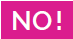and. I’ll spend the rest of the article exploring those.

Without loss of generality, I assert that an arch’s sole reason for existence is to support a bridge carrying a railway over a canal.

##You can have an arch without shear forces……as long as the railway and canal are perpendicular

It’s perfectly possible to build an arch without shear forces, as long as the railway travels at right angles to the canal.

This gives you the simplest type of arch—the archetype, if you like. The bricks are laid in horizontal rows, each row tilted slightly further towards the centre of the canal than the row below it, so that from the viewpoint of a barge, you see something like a semicircle or an ellipse as you approach the bridge, like this: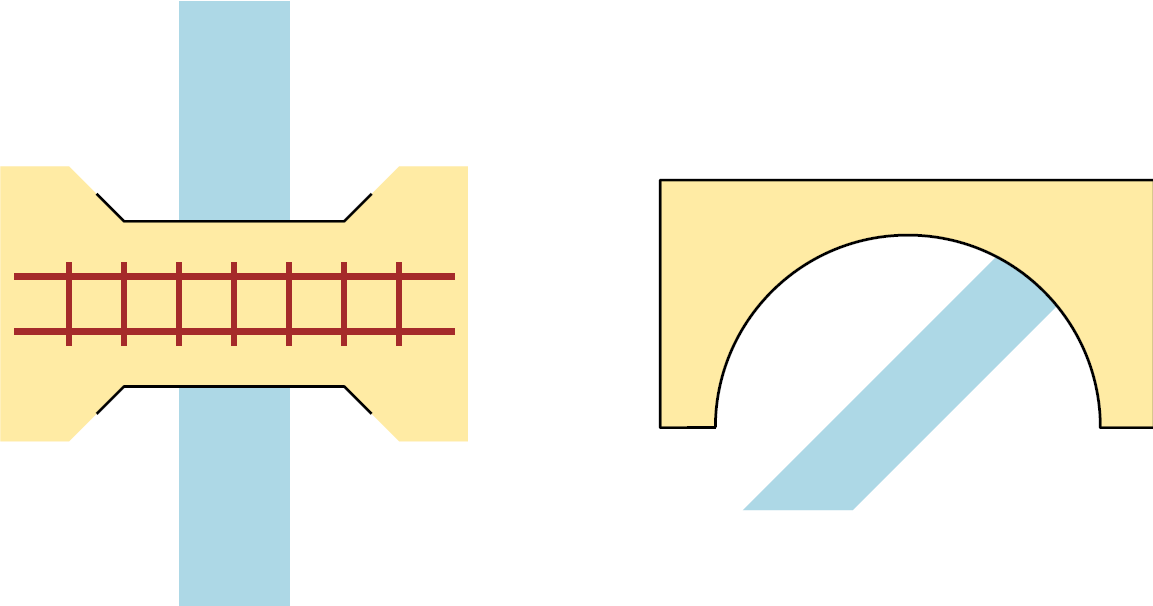So what happens with the forces here? The weight of the railway acts in vertical planes parallel to the tracks.

It’s easiest to see if we unwrap the cylinder to get a rectangle; the forces become straight lines and the bricks are, quite naturally, laid in lines at right angles to the forces: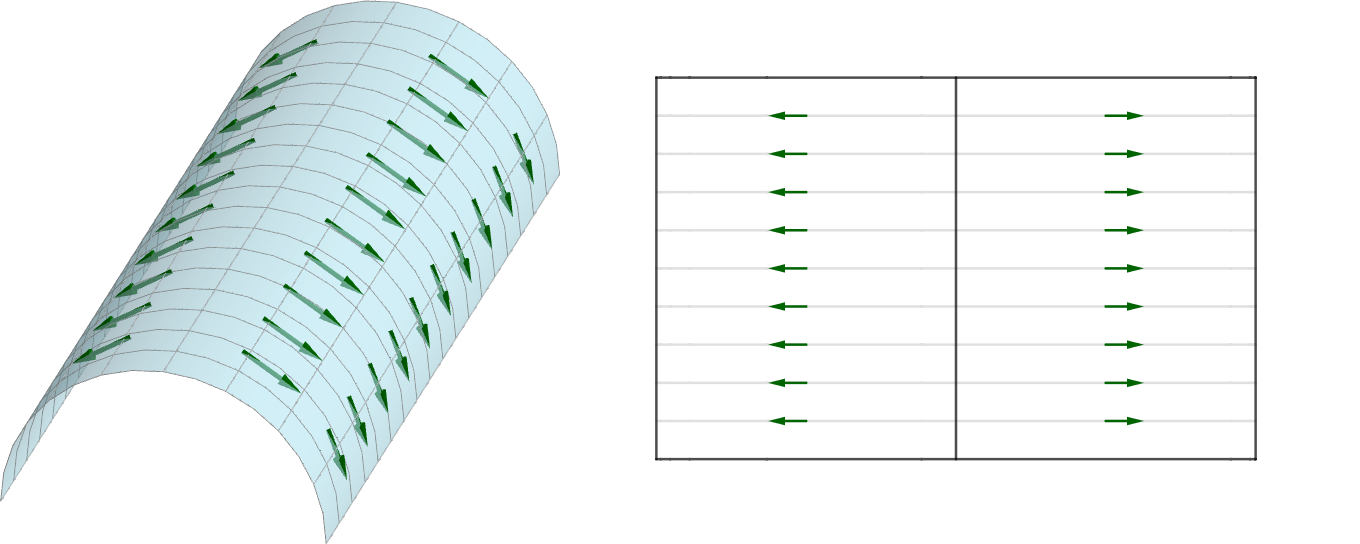Wrap it back up, and there’s your arch. But what if the canal and railway aren’t perpendicular?

##You can’t have an arch without shear forces……if you insist on laying the bricks in rows

For a long time, there was only one reasonable solution to having a track cross a river at anything other than right angles: move the track so that it does cross the river at a right angle. With a path or a road that’s, at worst, a bit annoying. Railways, though, don’t do sharp bends: you have to cross the canal at an arbitrary angle. So what’s the problem with that? Why not just build an arch with horizontal bricks like before? The problem is, your arches fall down. The strength of the original arch comes from the two halves leaning against each other, all the way along. If you simply chop off a diagonal from the ends of your arches, there’s nothing holding up the bits that remain—the red areas below: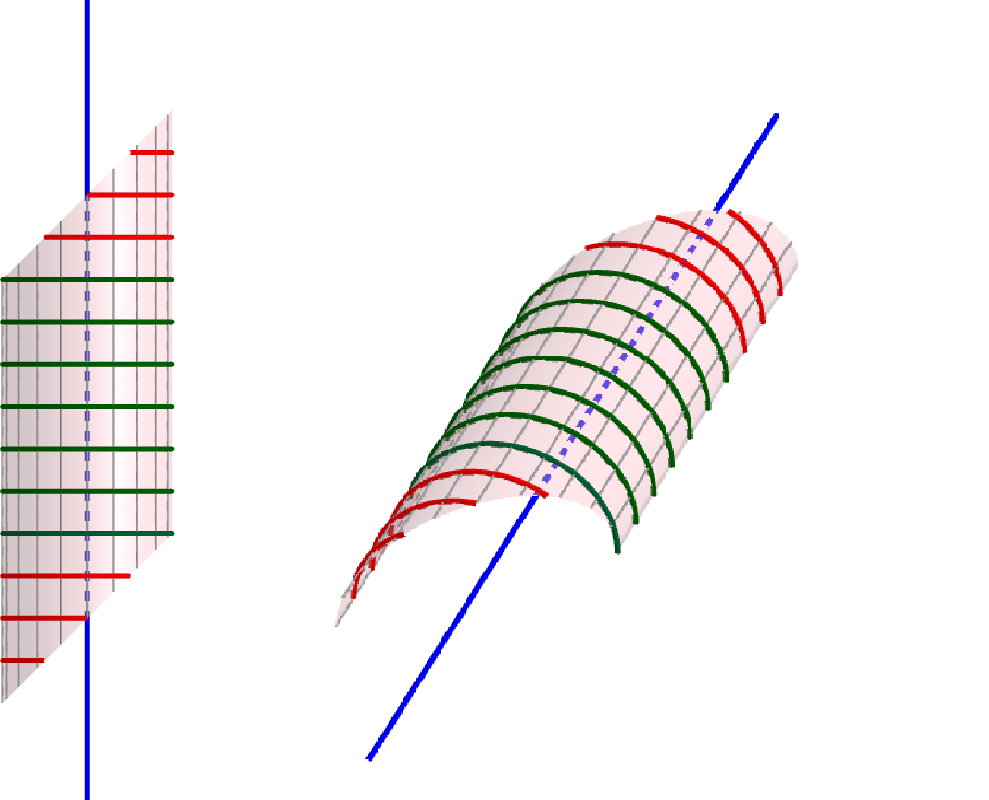Fine, then (I hear you say): why not start with a row of bricks perpendicular to the railway? That’s a good idea. Let’s see what happens by doing another unwrapping experiment.

Start with a semi-circular cylinder—you can cut a toilet roll tube in half lengthways if you want to—and chop the ends diagonally (say at 30\degree) to represent the direction of the railway line. Draw grey lines parallel to these end faces along the tube, representing the weight of the railway: this acts in vertical planes parallel to the tracks.

You should have something like this:Before you unroll it, predict what shape you’ll get when you do. I was surprised.

The answer is…Now let’s draw the rows of bricks on.

Find the middle of the top of the arch—midway between the flat edges, and halfway along a line parallel to them. Draw a line through this point, perpendicular to the ends—then as many lines as you like parallel to these representing your bricks:

Hopefully you’ll notice at least one of two things when you fold it back up, or inspect the drawing above:

• the lines of bricks reaching the ground do so at an angle, rather than parallel to the canal; and
• the lines of bricks are not perpendicular to the forces except along the first line you drew.

The first point explains why some bridges have bricks at a diagonal. The second of these explains why the answer to the question was ‘no’—there is shear almost everywhere in this type of bridge, and you need to do some clever engineering to counteract it. (‘Do some clever engineering’ is code for ‘build more bridge’.) More to the point, the steeper the angle between the canal and the railway, the more engineering you have to do, and you reach a point where it becomes impossible.

But surely there’s a way to cross a river at a steep angle? Step aside, engineers: we’re going to do some maths.

##You can have a bridge without shear forces……if you change the shape of the bricks

At this point, we meet the hero of the story: Edward Sang, who is best known for constructing state-of-the-art logarithmic tables and for showing how a spinning top can prove that the Earth rotates.

Around the end of 1835, he presented a proposal to the magnificently named Society for the Encouragement of the Useful Arts, explaining how he would build a bridge. Naturally, he came up with the most mathematician-esque suggestion possible: he redefined the meaning of ‘brick’.

If we want the joins between the stones to be perpendicular to the weights exerted on them, the bricks can’t be rectangular—they need to have curved surfaces. Moreover, there are very precise constraints on the curves where these bricks meet, which I’ll call the joining curves. At every point, they must:

1. lie in a plane perpendicular to the weight force exerted there; and
2. lie on the surface of the arch.

We can accomplish that by cunning use of vector calculus. Any curve that lies in two surfaces is (locally) perpendicular to the surfaces’ normal vectors—which in this case are the weight vector at the point and the normal vector to the surface. To find the direction of the curve, we can take the vector product of these. This is not precisely the way that Sang did it; it’s a way I can understand it.

First, we’re going to set up a system of coordinates. Let the $x$-axis (with a unit vector $\mathbf{i}$) run horizontally, perpendicular to the canal, the $y$-axis (with a unit vector $\mathbf{j}$) run horizontally along the centre of the canal, and the $z$-axis (with a unit vector $\mathbf{k}$) be vertical. Here’s what it looks like:The weight forces lie in vertical planes parallel to the tracks, as before. If our railway runs at an angle $\theta$ to the $x$-axis, these planes satisfy \begin{equation*} y = x \tan(\theta) + y_0, \end{equation*} where $y_0$ is a different constant for each plane. To save on typesetting, I’ll let $\lambda = \tan(\theta)$.

The next question is, what shape should our bridge’s cross-section be? Until now, we’ve only looked at semicircular cylinders, but these are not practical for canals: typically, we might want a railway to cross about three metres above a canal that’s eight metres wide. An arc of a circle is no good (there’s not enough clearance for someone to walk—or, these days, cycle—along the towpath), so our best bet is a semi-ellipse.

A semi-elliptical cylinder with width $2a$ and height $b$ following our coordinate system satisfies the equation \begin{equation*} \frac{x^2}{a^2} + \frac{z^2}{b^2} = 1, \end{equation*} or, if we set $e=b/a$,

$$e^2x^2 + z^2 = b^2. \tag{1}$$

Note that $z$ is positive everywhere in our arch, so it can be rewritten as a function of $x$. We won’t do that explicitly, because it’s unnecessarily ugly. However, we will need to know $\mathrm{d} z/ \mathrm{d} x$ in a few paragraphs, so let’s differentiate implicitly here: \begin{equation*} 2e^2 x + 2z \frac{\mathrm{d}z}{\mathrm{d}x} = 0, \quad \text{so} \quad \frac{\mathrm{d}z}{\mathrm{d}x} = -e^2 \frac{x}{z}. \end{equation*} Any point on the surface can then be described by the position vector $x \mathbf{i} + y \mathbf{j} + z(x) \mathbf{k}$. This is important, because we can then find the curves where the force planes intersect the cylinder: we know that $y = \lambda x + y_0$, so our forces follow the curve \begin{equation*} \mathbf{r}(x) = x \mathbf{i} + (\lambda x + y_0)\mathbf{j} + z(x)\mathbf{k}. \end{equation*} The direction of the weight force is parallel to the derivative of this curve at any point. If we differentiate $\mathbf{r}(x)$ with respect to $x$, we get \begin{equation*} \frac{\mathrm{d}\mathbf{r}(x)}{x} = \mathbf{i} + \lambda \mathbf{j} + \frac{\mathrm{d}z(x)}{\mathrm{d}x} \mathbf{k} = \mathbf{i} + \lambda \mathbf{j} – e^2 \frac{x}{z} \mathbf{k}. \end{equation*} Since we’re only interested in the direction, we can multiply through by $z$ to get \begin{equation*} \mathbf{F} \propto z \mathbf{i} + \lambda z \mathbf{j} + -e^2 x\mathbf{k}. \end{equation*} That’s one of the ingredients for our vector product! The other is the normal vector to the surface. That lies in a plane perpendicular to the axis, so it turns out to be the normal vector to the ellipse in (1); with a little work, we get \begin{equation*} \mathbf{n} = e^2 x \mathbf{i} + z \mathbf{k}. \end{equation*} The vector product is \begin{equation*} \mathbf{n} \times \mathbf{F} = -\lambda z^2 \mathbf{i} + (z^2 + e^4 x^2)\mathbf{j} + \lambda e^2 xz \mathbf{k}, \end{equation*} and this is the direction vector of our joining curve at any given $x$ value along it. We eventually want our joining curve in the form $\mathbf{R}(x)$.

Again, we can multiply by anything convenient here, since we only care about the direction: if we pick $-1/(\lambda z^2)$, we get a direction vector of \begin{equation*} \mathbf{i}- \frac{1}{\lambda}\left(1 + \frac{e^4 x^2}{z^2}\right) \mathbf{j} – \frac{e^2 x}{z} \mathbf{k}. \end{equation*} Why would we make it messier like this? Because by making the $\mathbf{i}$ component 1, we have a natural expression for $\mathrm{d}R/\mathrm{d}x$, which we can then integrate. The $\mathbf{i}$ component is trivial—ignoring constants of integration until later, that integrates to $x$. The $\mathbf{k}$ component isn’t much harder: it’s in the form we got from differentiating equation (1), so we know it integrates to $z$, as we would hope. The $\mathbf{j}$ component is not so straightforward, but it’s still possible. To work out \begin{equation*} -\frac{1}{\lambda} \int \left [ 1 + \frac{e^4 x^2}{z^2} \right] \mathrm{d}x, \end{equation*} first (counterintuitively) substitute for the $x^2$: \begin{align*} -\frac{1}{\lambda} \int & \left [ 1 + \frac{e^2 \left(b^2 – z^2\right)}{z^2} \right ] \mathrm{d}x \\ &= -\frac{1}{\lambda} \int \left[ \left(1-e^2\right) + \frac{e^2 b^2}{z^2} \right ]\mathrm{d}x. \end{align*} Now we can substitute for the $z^2$,\begin{equation*} = -\frac{1}{\lambda} \int \left[ \left(1-e^2\right) + \frac{ e^2 b^2}{b^2 – e^2 x^2} \right] \mathrm{d}x, \end{equation*} and integrate, \begin{equation*}= -\frac{1}{\lambda} \left[ \left( 1 – e^2 \right)x + e b\ \mathrm{arctanh} \left( \frac{ex}{b}\right)\right]. \end{equation*} So, finally we get that our curves have the form \begin{align*} \mathbf{R}(x) = \; & \mathbf{r}_0 + x \mathbf{i} \\ & – \frac{1}{\lambda}\left[ \left( 1 – e^2 \right)x + e b\ \mathrm{arctanh} \left( \frac{ex}{b}\right)\right] \mathbf{j} + z \mathbf{k}, \end{align*} where $\mathbf{r}_0$ is any point on the surface of the arch. Written like that, it looks like a bit of a mess. But look below what happens when you plot it—it’s glorious!

And that brings us the Liverpool–Leeds canal, which the west coast mainline crosses at a precipitous $53^\circ$ off of square, near Chorley in Lancashire (///bottle.cuts.space, if you’re a what3words aficionado). And, in the 1840s, they built bridge 74A according to Sang’s principles. As far as I can make out, the masons building bridge 74A didn’t do the calculus. They rolled up paper on a model and drew ellipses in the right place, which I have to concede is a pretty decent pre-WolframAlpha solution to the problem.

You can see this splendid piece of engineering below. If you visit and stop on the towpath, for example to admire the brickwork and take photos, passers-by will engage you with their understanding of the bridge’s history—it was hand-carved by French stonemasons, there’s another one just like it south of Bolton station, probably the same hands—and share your observation that it’s a good bridge.

Next time you’re walking under a bridge, look up. If it’s a good bridge, stop passers-by and explain the principles to them. They might appreciate it.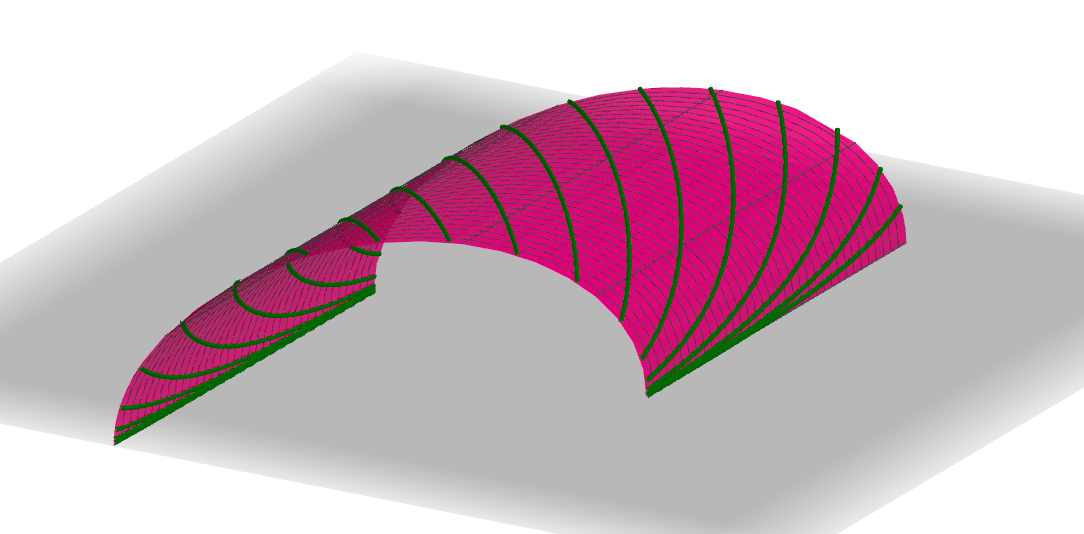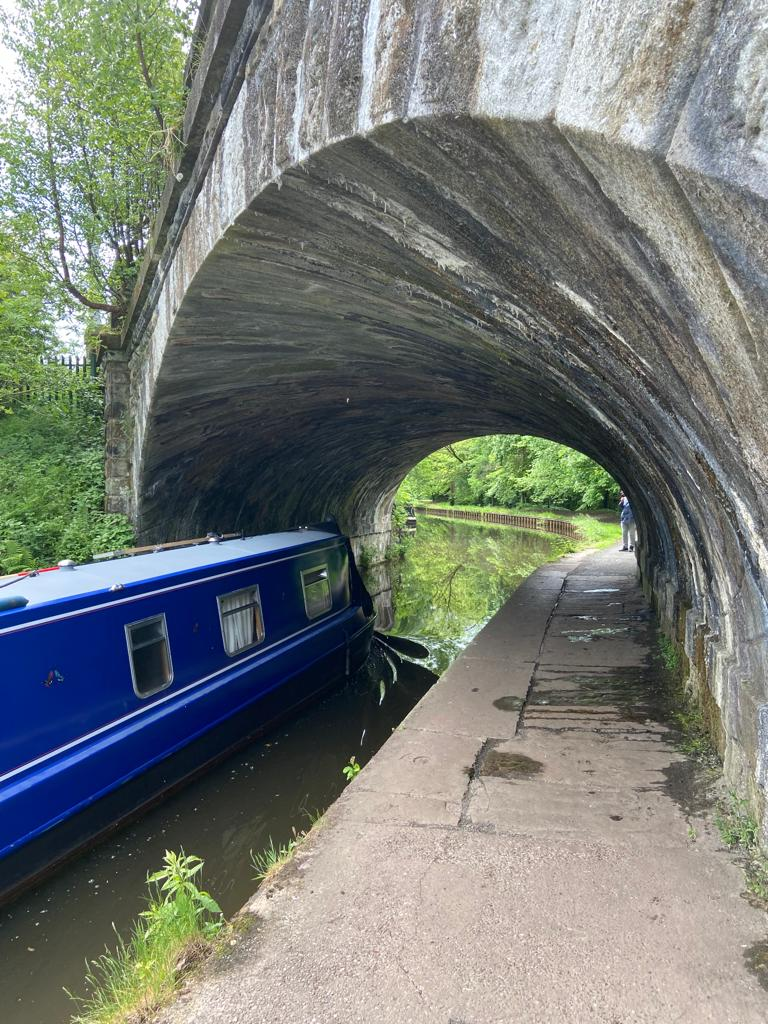Bridge 74A on the Liverpool–Leeds canal, constructed like the figure above.Colin is the author of Cracking Mathematics and The Maths Behind, written to prove that he has nothing to prove (by contradiction).